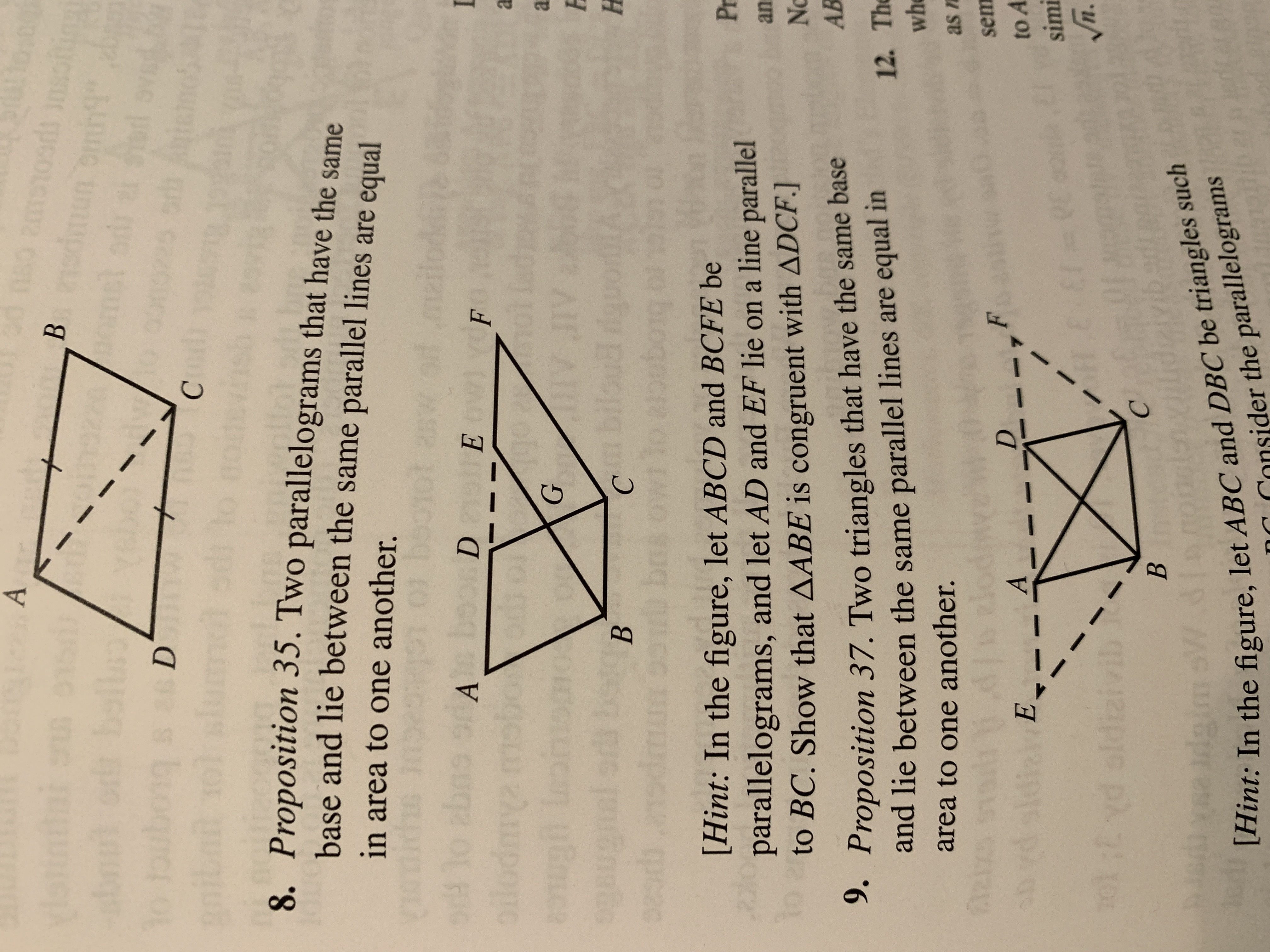ABododra sionbellborg29Dsbu sd8. Proposition 35. Two parallelograms that have the samebase and lie between the same parallel lines are equalin area to one another.91bos DSib orot asw9lo abosoilod mm0 lohiomAYFEaEdoy199GAV JIVeonuenl edsbiloua dgpo930Bodma029d0u brs owiio 2Hint: In the figure, let ABCD and BCFE beparallelograms, and let AD and EF lie on a line parallel10 to BC. Show that AABE is congruent with ADCF.]9. Proposition 37. Two triangles that have the same basePranNoAB12. Thewhand lie between the same parallel lines are equal inarea to one another.aaicas nelodsemFDADdiaiEto AsimiEED= VEVn101:Eyd oldiziviCBdai sW[Hint: In the figure, let ABC and DBC be triangles suchonsider the parallelograms

Question

Prove the indicated result of Proposition 35 in question #8 that pertains from Book 1 of Euclid’s Elements.help_outlineImage TranscriptioncloseA B od odra sion bell borg 29 D sb u sd 8. Proposition 35. Two parallelograms that have the same base and lie between the same parallel lines are equal in area to one another. 91 bos D Sib or ot asw 9lo abos oilod m m0 lohiom A YF E a Edoy 199 G AV JIV eonue nl eds biloua dgpo 930 B odma 029d0 u brs owiio 2 Hint: In the figure, let ABCD and BCFE be parallelograms, and let AD and EF lie on a line parallel 10 to BC. Show that AABE is congruent with ADCF.] 9. Proposition 37. Two triangles that have the same base Pr an No AB 12. The wh and lie between the same parallel lines are equal in area to one another. aaic as n elod sem F D A D diaiE to A simi EED= VE Vn 101:Eyd oldizivi C B dai sW [Hint: In the figure, let ABC and DBC be triangles such onsider the parallelograms fullscreen
Step 1

Consider the given figure:

Step 2

Now, in triangle ABC and DCF :

AB=CD  (since, ABCD is a parallelogram)

∠ABE = ∠DCF  (since, ∠BAD= ∠CDF and Angle ∠AEB = angle ∠DFC)

BE=CF  (since, BEFC is a parallelogram)

Thus, by SAS property:

∆ABC∆DCF

Step 3

Therefore,

Ar (∆ABC) = Ar (∆DCF)

Ar (ABGD)+ Ar (DGE) = Ar (EFCG) + Ar (DGE)

Ar (ABGD) = Ar (EFCG)

Now, add the area of triangle BGC to both sides:

Ar (ABGD)+ Ar (BGC) = Ar (EFCG) + Ar (BGC)

Ar (ABCD) = Ar (BCF...

Want to see the full answer?

See Solution

Want to see this answer and more?

Our solutions are written by experts, many with advanced degrees, and available 24/7

See Solution
Tagged in

Math